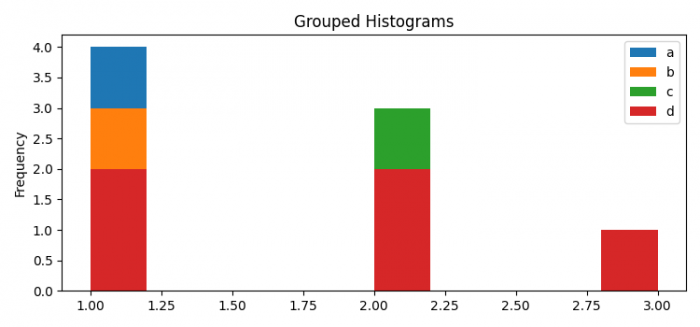# How to add legends and title to grouped histograms generated by Pandas? (Matplotlib)

MatplotlibPythonData Visualization

To add legends and title to grouped histograms generated by Pandas, we can take the following steps −

• Set the figure size and adjust the padding between and around the subplots.
• Create a Pandas dataframe with "a", "b", "c" and "d" keys.
• Plot data frame with kind="hist"
• Set a title for the axes.
• To display the figure, use show() method.

## Example

from matplotlib import pyplot as plt
import pandas as pd

plt.rcParams["figure.figsize"] = [7.50, 3.50]
plt.rcParams["figure.autolayout"] = True

df = pd.DataFrame({'a': [1, 1, 1, 1, 3],
'b': [1, 1, 2, 1, 3],
'c': [2, 2, 2, 1, 3],
'd': [2, 1, 2, 1, 3],
})
df.plot(kind='hist')
plt.title("Grouped Histograms")

plt.show()

## Output